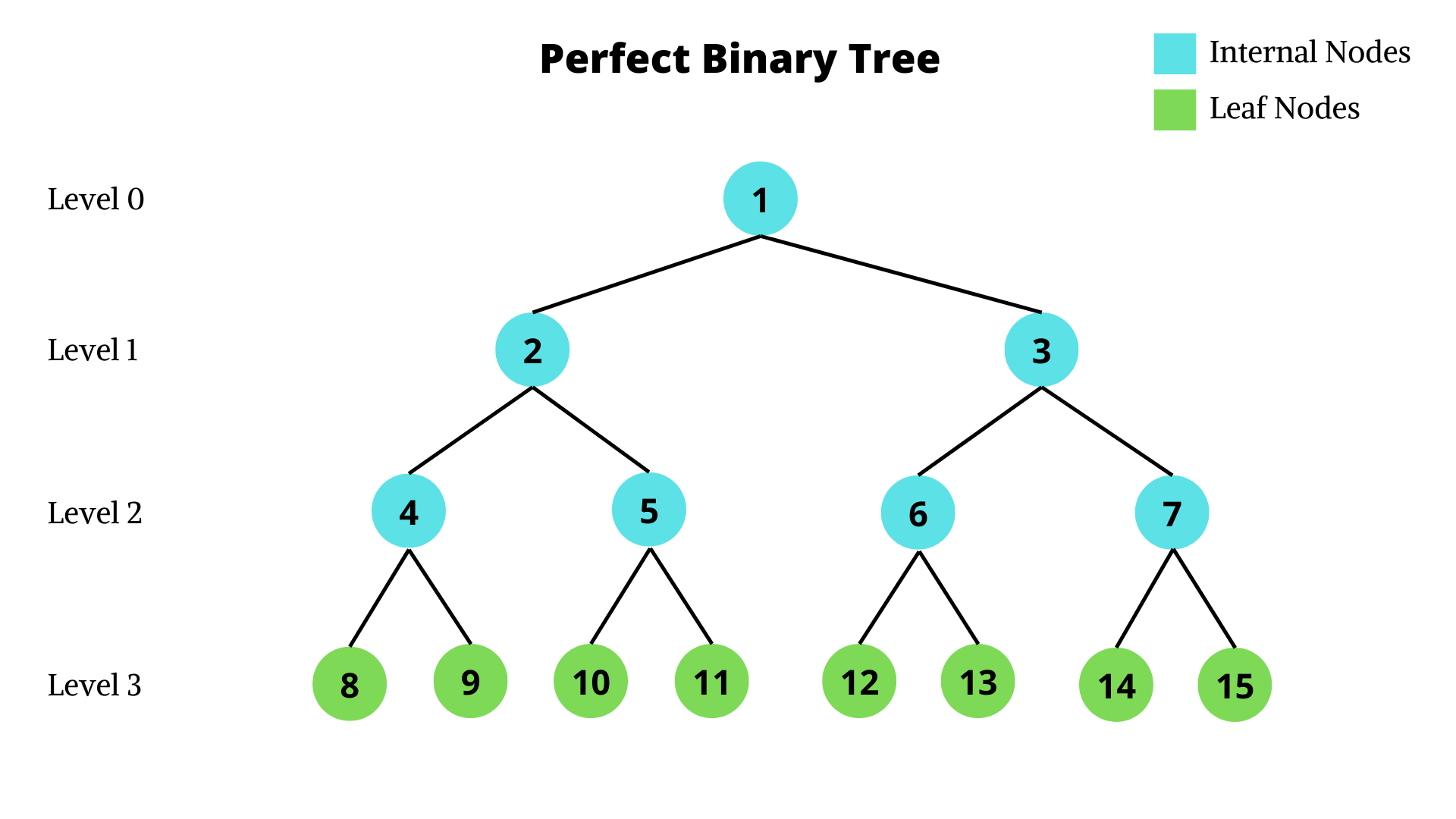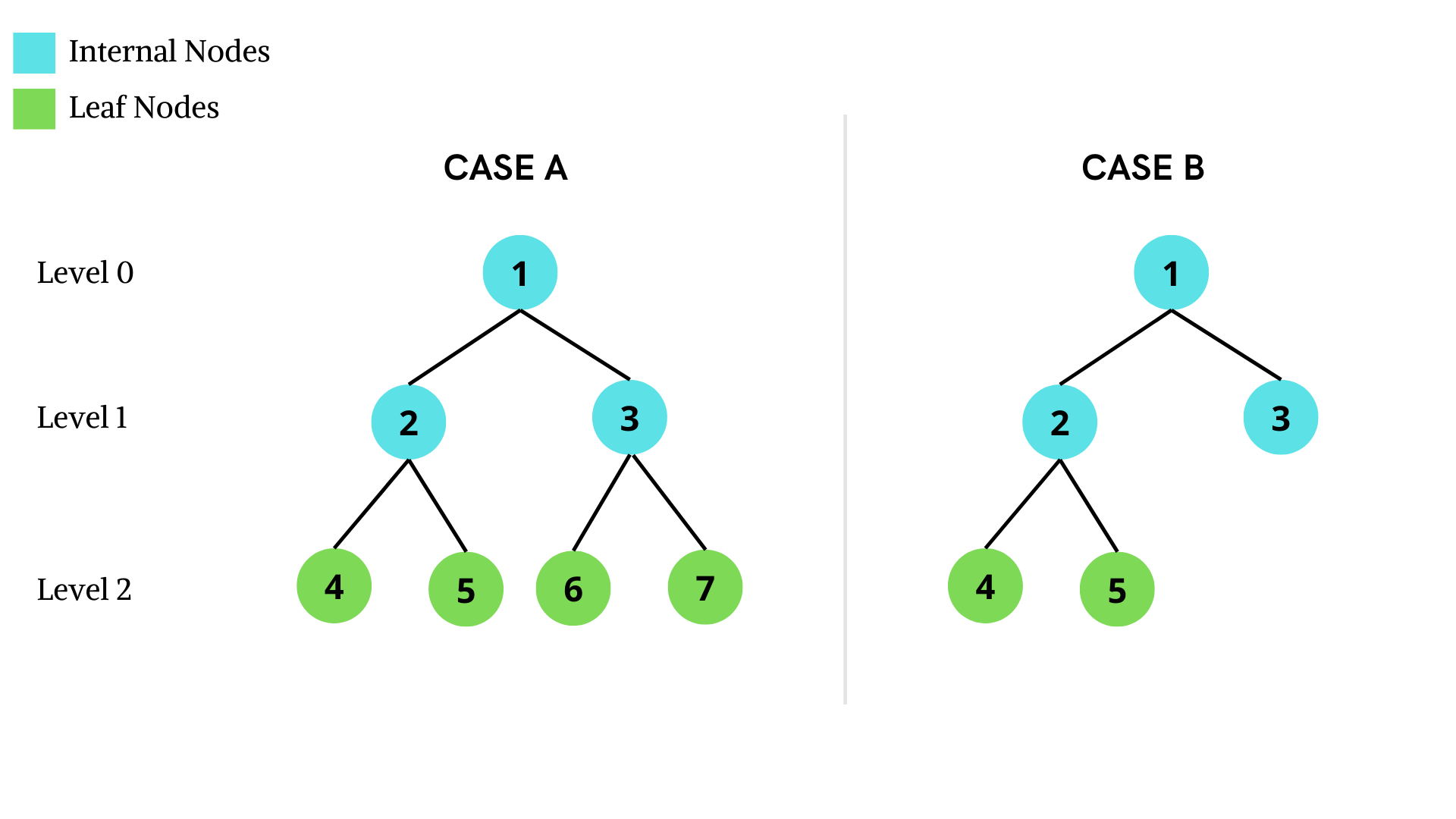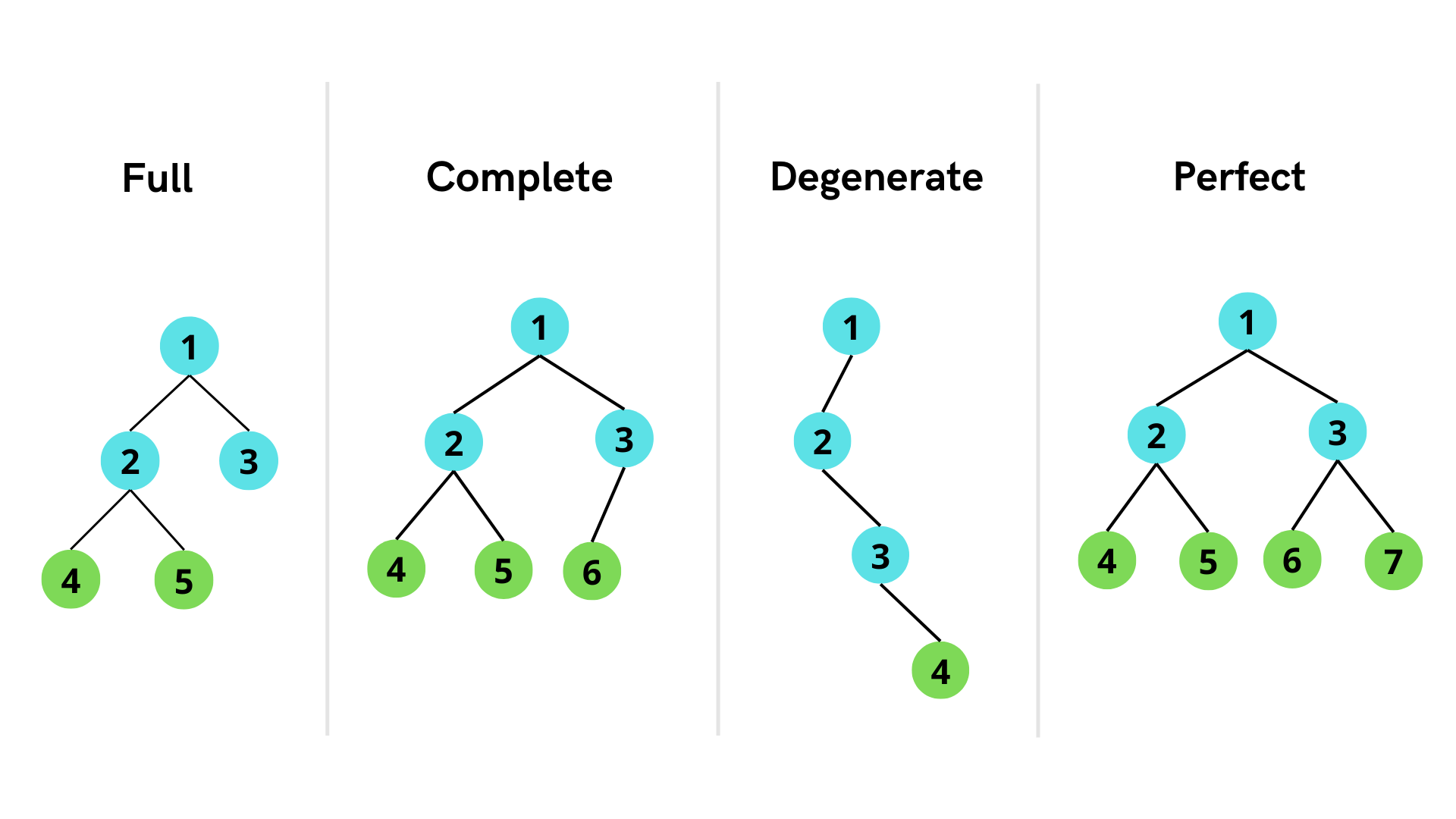×

Search anything:

# Perfect Binary Tree

#### Problems on Binary Tree AlgorithmsGet this book -> Problems on Array: For Interviews and Competitive Programming

Perfect Binary Tree is one of the types of Binary Tree data structure. In this article, we will learn about the Perfect Binary Tree and its properties. We will also see how it differs from other types of Binary Tree.

### Table of Content

1. Introduction
2. Properties
3. Program
4. Comparison

# Introduction

A Perfect Binary Tree is a binary tree in which all the internal nodes have 2 children and the leaf nodes are at the same level.In the above given diagram, all the internal nodes have 2 children each. All the leaf nodes are all at one level which makes it the last level.

# Properties

1. The total number of nodes (n) of a Perfect Binary Tree of height h is given by the equation, n = 2^(h + 1) – 1. In the above example, the tree is of height 3 and the total number of nodes are 15 (2^(3+1) - 1).

2. Height (h) of a given Perfect Binary Tree with n nodes is given by, h = log2(n + 1) – 1.

3. Number of leaf nodes(l) in a Perfect Binary Tree of height h is given by, l = 2^h. In the given example, the number of leaf nodes is 8 (2^3).

4. Number of internal nodes (i) in a Perfect Binary Tree of height h is given by, i = 2^h - 1. It can be seen in the example given above that the number of internal nodes are 7 (2^3 - 1).

5. Relation between internal nodes and leaf nodes, i = l - 1.

# Program

In order to check if a given Binary Tree is Perfect or not, we have to check if all the internal nodes have 2 children. If a node has no children, we have to check it's level. If the node is not at the last level, the tree is not a Perfect Binary Tree.

#### Steps:

1. Construct a Binary Tree.
2. Find the depth on the Binary Tree. The height of any one leaf node will be sufficient for this problem since if it is a Perfect Binary Tree, all the leaf nodes will lie at the same level.
3. Traverse through the Binary Tree in an inorder manner.
4. At every node, check if it has two children. If a node has one child, the tree is not Perfect. Otherwise, check the left child and right child and return the answer.
5. If a node is a leaf node, check the level of the node. If the node is not at the depth, it is not Perfect.

#### Pseudocode:

``````FUNCTION isPerfectTree(node, level)
IF right and left child are null
IF level of node is equal to depth
RETURN true
ELSE
RETURN false
IF right and left child both are not null
CALL isPerfectTree(left child, level + 1) && isPerfectTree(right child, level + 1)
ELSE
RETURN false
``````

#### Java Implementation:

``````// define the nodes of the binary tree

class Node {
int val;
Node left;
Node right;

// constructor for Node class
Node(int num) {
val = num;
left = right = null;
}
}

// Binary tree construction and operations
class BinaryTree {
Node root;
int depth;

void findDepth(Node node) {
int level = 0;
while (node.left != null) {
node = node.left;
level++;
}
this.depth = level;
}

// function to check if a tree is a perfect binary tree
boolean isPerfectTree(Node node, int level) {
// in case the tree is empty, it is a Perfect binary tree
if (node == null)
return true;

// in case the node is a leaf node
if (node.left == null && node.right == null)
return level == depth;

// if the node has two children
if ((node.left != null) && (node.right != null))
return isPerfectTree(node.left, level + 1) && isPerfectTree(node.right, level + 1);

// in case the node has one child
return false;
}

// function to print the result
void printResult(Node node) {
if (isPerfectTree(node, 0))
System.out.println("The binary tree is a Perfect Binary Tree");
else
System.out.println("The binary tree is not a Perfect Binary Tree");
}

public static void main(String args[]) {

// construct the binary tree for case A
BinaryTree casea = new BinaryTree();
casea.root = new Node(1);
casea.root.left = new Node(2);
casea.root.right = new Node(3);
casea.root.left.left = new Node(4);
casea.root.left.right = new Node(5);
casea.root.right.left = new Node(6);
casea.root.right.right = new Node(7);

// find depth of the tree
casea.findDepth(casea.root);

// print result for case A
casea.printResult(casea.root);

// construct the binary tree for case B
BinaryTree caseb = new BinaryTree();
caseb.root = new Node(1);
caseb.root.left = new Node(2);
caseb.root.right = new Node(3);
caseb.root.left.left = new Node(4);
caseb.root.left.right = new Node(5);

// find depth of the tree
caseb.findDepth(caseb.root);

// print result for case B
caseb.printResult(caseb.root);

}
}
``````

#### Output:

The binary tree is a Perfect Binary Tree
The binary tree is not a Perfect Binary Tree

#### Explanation:In case A, all the internal nodes have 2 children each and the leaf nodes are present at the same level. Hence, it is a Perfect Binary Tree. However, in case B, the leaf nodes are present at level 1 and level 2, hence, it is not a Perfect Binary Tree.

Time complexity: Time taken to calculate depth O(h) + Time taken to traverse the tree O(n)
Auxiliary Space: O(n) for call stack

# Comparison

Full Complete Degenerate Perfect
All internal nodes have 2 children Internal nodes can have 1 or 2 children. Although, only one internal node can have 1 child. All internal nodes only have 1 child All internal nodes have 2 children
Leaf nodes can be present at any level Leaf nodes present at last and second last level Leaf nodes present at the last level Leaf nodes are present at the same level
The maximum number of nodes 2^(h +1) - 1, minimum number of nodes 2^h + 1 The maximum number of nodes 2^(h +1) - 1, minimum number of nodes 2^h Total number of nodes h + 1 Total number of nodes 2^(h +1) - 1With this article at OpenGenus, you must have the complete idea of Perfect Binary Tree.#### Astha Jain

Astha Jain is a BE student of Information Technology (IT) at Savitribai Phule Pune University.